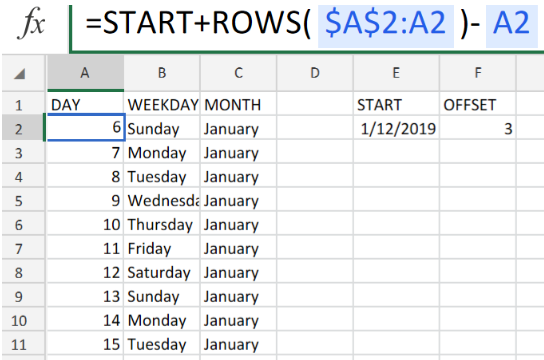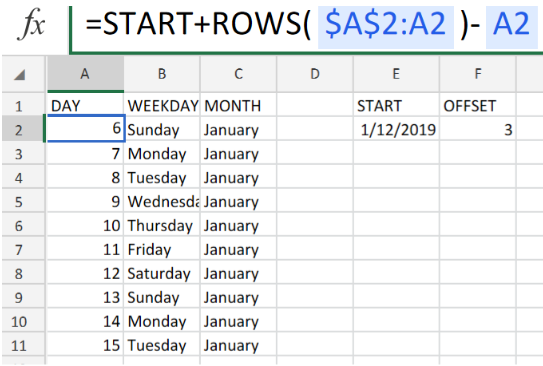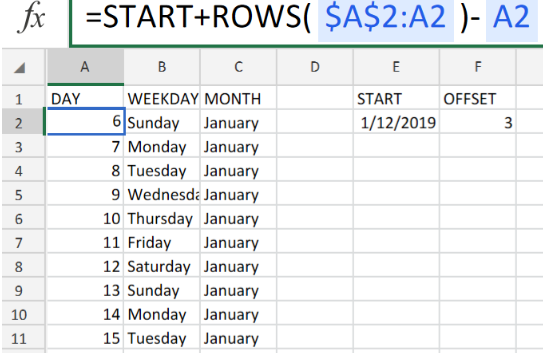Get instant live expert help with Excel or Google Sheets“My Excelchat expert helped me in less than 20 minutes, saving me what would have been 5 hours of work!”

#### Post your problem and you’ll get Expert help in seconds.

Your message must be at least 40 characters
Our professional Expert are available now. Your privacy is guaranteed.

# How to Use the Dynamic Date List Function in ExcelFigure 1. Dynamic Date List in Excel.

In order to set up a dynamic date list in Excel, we are going to use a formula syntax that allows us to automatically update it according to our specified parameters.

## Formula Syntax

`=start+ROWS(exp_rng)-1-offset`

• start refers to our specified range of dates
• offset refers to the days which are before the date of start.

Excel dates are basically serial numbers that are displayed in recognized date format. Thus, we can carry out mathematical operations on Excel dates to determine days in the past or future.

## How to Use the Excel Dynamic Date List Function.

In our worksheet example below, the formula in cell A2 begins with our start date, and increases the date by 1 with an expanding range within the ROWS formula.Figure 2. Dynamic Date List in Excel.

The row count is increasing by 1 at each new row while the range expands.

To ensure that the date is not increasing in the first row, the Dynamic Date List formula subtract 1 from each value.Figure 3. Final Result.

## Instant Connection to an Expert through our Excelchat Service:

Our live Excelchat Service is here for you. We have Excel Experts available 24/7 to answer any Excel questions you may have. Guaranteed connection within 30 seconds and a customized solution for you within 20 minutes.

### Did this post not answer your question? Get a solution from connecting with the expert.Another blog reader asked this question today on Excelchat:
Related blogs
Solution examplesI need to create a pivot table that sums visits per day by provider: Date...Visits....Provider So, I can ask...how many visits did provider X have on day Y?
Solved by F. J. in 34 minscould someone breakdown this formula for me :D =ArrayFormula(IF(D\$5>=ROWS(B\$5:B5),INDEX(Sheet1!C\$6:C\$21,SMALL(IF(Sheet1!D\$6:D\$21=\$E\$5,ROW(Sheet1!C\$6:C\$21)-ROW(Sheet1!C\$6)+1),ROWS(B\$5:B5))),""))
Solved by C. C. in 40 minsDIVIDE NUMBER IN COLUMN A BY A FIXED NUMBER AND THEN SUBTRACT THAT TOTAL BY 1. HOW DO I COPY AND PASTE THIS FORMULA SO THAT IT APPLIES TO ALL OF THE ROWS?
Solved by A. U. in 40 mins## Subscribe to Excelchat.coAnother blog reader asked this question today on Excelchat: